## Calculus-Derivatives

Version 1.2.1.0 (4.55 MB) by
Interactive module that introduces fundamentals of derivatives including the product and chain rule as presented in Calculus I courses

Updated 27 Jan 2023

From GitHub

# Calculus Derivatives

Curriculum Module
Created with R2021a. Compatible with R2021a and later releases.

## Description

This curriculum module contains interactive live scripts and MATLAB® apps that teach basic concepts of derivative calculus. There is a focus on numerical approximation and graphical representation as tools for understanding the concepts of calculus.

The derivatives module is divided into four scripts and a practice app. The first covers the limit definition of the derivative and includes several motivating examples for the study of derivatives. The second covers derivatives of powers and the linearity of derivatives working from the limit definition of the derivative, including an application to parabolic motion. The third covers derivatives of exponentials, natural logarithms, sines and cosines as well as discussing higher order derivatives. Applications include population growth and oscillatory behavior. The fourth script presents visualizations of the product and chain rule for derivatives as well as randomly generated practice problems. Applications include related rates and the quotient rule for derivatives. The Calculus Flashcards app allows users to select the types of derivative (or integral) rules they wish to practice, generates randomized problems, and tracks their progress within a session.

These lessons can be used as part of a lecture, as activities in an instructional setting, or as interactive assignments to be completed outside of class. All interactive exercises offer feedback while reflection questions are more open-ended and do not include solutions in this module.

Loading the project file, Derivatives.prj, will add all of the required scripts to the path and open a navigation page. The instructions inside the live scripts will guide you through the exercises, examples, and activities. Get started with each live script by running it one section at a time. To stop running the script or a section midway (for example, when an animation is in progress), use the Stop button in the Run section of the Live Editor tab in the MATLAB Toolstrip.

## Prerequisite Domain Knowledge

This module assumes a knowledge of functions that is standard in precalculus course materials regarding powers, exponentials, absolute values, logarithms, sines, cosines, rational functions, and asymptotes. In addition, this module assumes basic fluency with limits in discussing the limit definition of the derivative.

## Suggested Prework

MATLAB Onramp – a free two-hour introductory tutorial that teaches the essentials of MATLAB.

## Details

An interactive script that facilitates exploration of the limit definition of the derivative and the relationship between slopes and derivatives.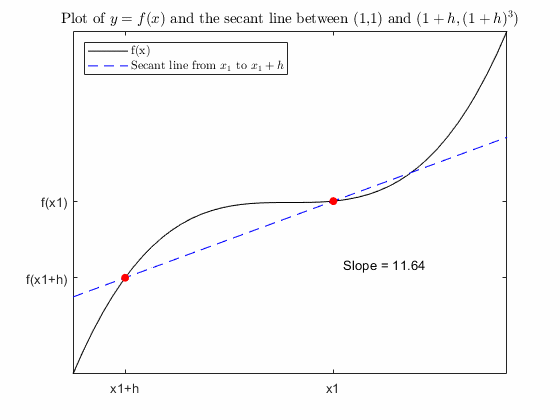Learning Goals:

• Explain the limit definition of the derivative and its relationship to secant lines
• Identify the sign of the derivative at a point as positive, negative, or zero based on the graph of a function
• Identify points where the derivative of a continuous function does not exist and explain why with reference to the definition of the derivative
• Recognize f'(x), df/dx, and d/dx[f(x)] as equivalent notation for the derivative of f with respect to x

An interactive script that facilitates exploration of the results of applying the limit definition of the derivative to powers to identify the power rule for derivatives. After introducing the linearity rules, there is the opportunity to generate and check random problems to practice applying the linearity and power rules for derivatives and an application to parabolic motion.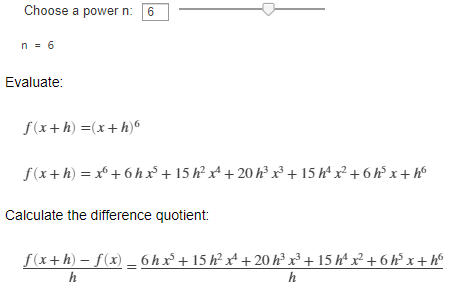Learning Goals:

• Explain why the limit definition of the derivative results in the power rule for derivatives
• Compute the derivatives of linear combinations of powers by hand
• Apply derivatives to solve practical questions

An interactive script that facilitates exploration of the derivatives of sines, cosines, exponential functions, natural logarithms, higher order derivatives, and unit analysis of derivatives.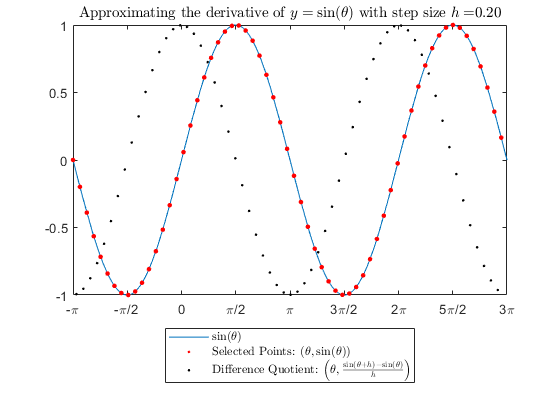Learning Goals:

• Determine the functional relationship of the derivatives of sines, cosines, exponentials, and natural logarithms
• Explain the pattern in the derivatives of sin(ax), cos(ax), and exp(ax) for constant values of a and that the derivative of ln(ax) does not fit the simple pattern
• Apply the correct units to derivatives of any order
• Explore simple first and second order differential equations that describe physical systems to create mathematical models of the system

An interactive script that presents visualizations of the chain rule and product rule as well as the opportunity to practice derivatives.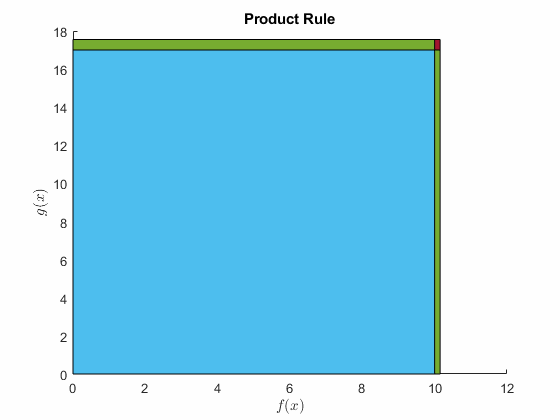Learning Goals:

• Explain why the chain rule is d/dx[f(g(x))] = f'(g(x))*g'(x)
• Apply the chain rule to calculate derivatives
• Explain why the product rule is d/dx[f(x)*g(x)] = f(x)*g'(x)+f'(x)*g(x)
• Apply the product rule to calculate derivatives
• Fluently recognize and apply the rules for computing derivatives

An interactive script that builds on the idea of approximating functions from tangent lines to Taylor Polynomials.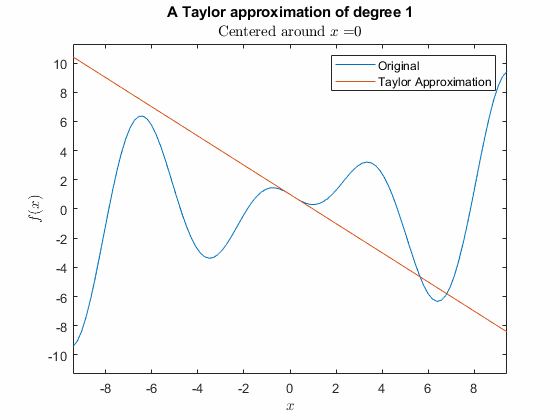Learning Goals:

• Identify and construct tangent lines.
• Identify and construct quadratic approximations.
• Use the structure of Taylor Polynomials to construct higher order approximations to differentiable functions.

A MATLAB app that allows the user to select among four different categories of derivative practice (simple derivatives, linear combinations of powers, product rule, and chain rule) or integral practice (simple integrals, definite integrals, substitution, and integration by parts) or any combination thereof. Variables can be restricted to x and t or varied randomly over a larger set of options. The solutions can be displayed immediately or multiple attempts at each problem can be recorded. Results are tracked by type of problem including correct solutions, incorrect solutions, and problems which are not even attempted before generating a new one.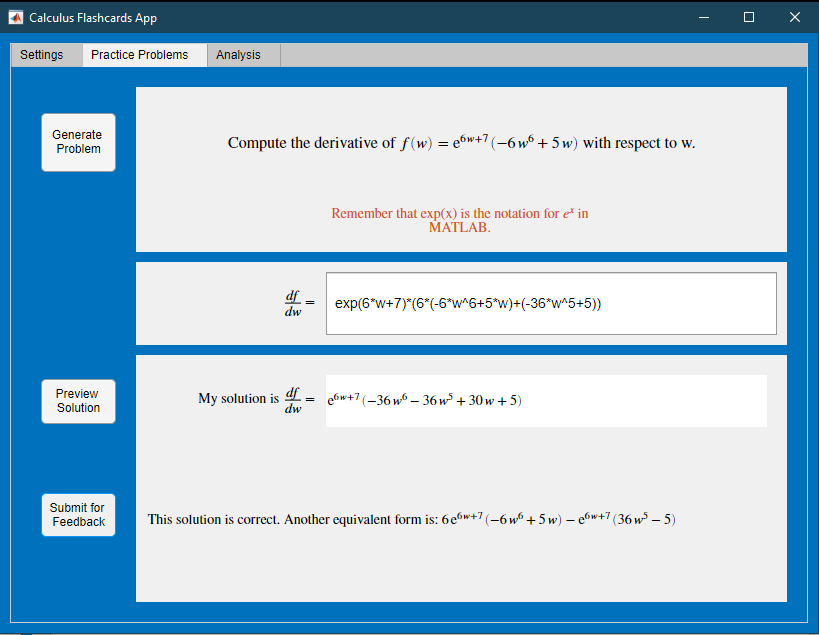Learning Goals:

• Computational fluency with common functions and interactions including linearity, the product rule, and the chain rule.

## Setup

### MATLAB®

1. Ensure that you have MATLAB R2021a or newer installed.
2. Download `CalculusFlashcards.mlapp` or download and unzip the entire repository.
3. Right-click the app in MATLAB and select run or double click on the live script (.mlx file) that you wish to run.

### MATLAB Online™

1. Download `CalculusFlashcards.mlapp` or download and unzip the entire repository.
2. Drag and drop `CalculusFlashcards.mlapp` into the Current Folder in MATLAB Online.
3. Right-click the `CalculusFlashcards.mlapp` and select run or double click on the live script (.mlx file) that you wish to run.

## Products

MATLAB®, Symbolic Math Toolbox™, Curve Fitting Toolbox™

The license for this module is available in the LICENSE.md file in this GitHub repository.

## Educator Resources

Have any questions or feedback? Contact the MathWorks online teaching team.

### Cite As

Emma Smith Zbarsky (2023). Calculus-Derivatives (https://github.com/MathWorks-Teaching-Resources/Calculus-Derivatives/releases/tag/v1.2.1), GitHub. Retrieved .

##### MATLAB Release Compatibility
Created with R2021a
Compatible with R2021a and later releases
##### Platform Compatibility
Windows macOS Linux
##### Communities
More Files in the  Distance Learning Community

### Community Treasure Hunt

Find the treasures in MATLAB Central and discover how the community can help you!

Start Hunting!

#### HelperFunctions

Version Published Release Notes
1.2.1.0

See release notes for this release on GitHub: https://github.com/MathWorks-Teaching-Resources/Calculus-Derivatives/releases/tag/v1.2.1

1.2.0

See release notes for this release on GitHub: https://github.com/MathWorks-Teaching-Resources/Calculus-Derivatives/releases/tag/v1.2.0

1.1.2

See release notes for this release on GitHub: https://github.com/MathWorks-Teaching-Resources/Calculus-Derivatives/releases/tag/v1.1.2

1.1.1

See release notes for this release on GitHub: https://github.com/MathWorks-Teaching-Resources/Calculus-Derivatives/releases/tag/v1.1.1

1.1.0

See release notes for this release on GitHub: https://github.com/MathWorks-Teaching-Resources/Calculus-Derivatives/releases/tag/v1.1.0

1.0.2

See release notes for this release on GitHub: https://github.com/MathWorks-Teaching-Resources/Calculus-Derivatives/releases/tag/v1.0.2

1.0.1

See release notes for this release on GitHub: https://github.com/MathWorks-Teaching-Resources/Calculus-Derivatives/releases/tag/v1.0.1

1.0.0

To view or report issues in this GitHub add-on, visit the GitHub Repository.
To view or report issues in this GitHub add-on, visit the GitHub Repository.